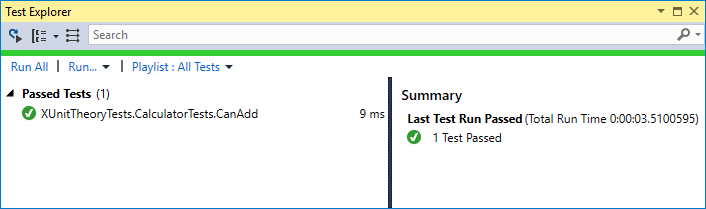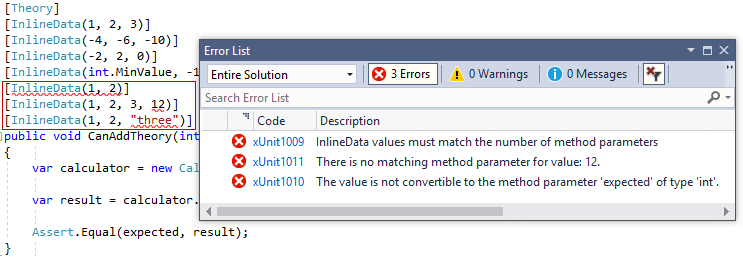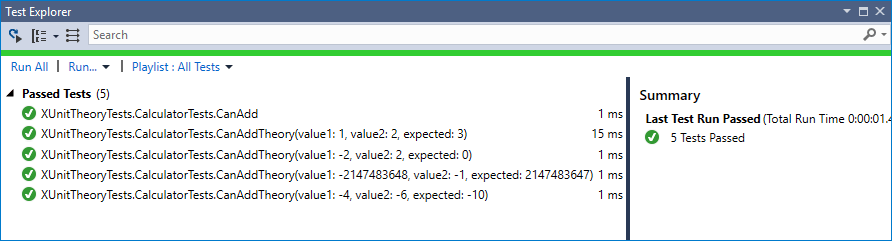# Creating parameterised tests in xUnit with [InlineData], [ClassData], and [MemberData]

Share on:

In this post I provide an introduction to creating parmeterised tests using xUnit's `[Theory]` tests, and how you can pass data into your test methods. I'll cover the common `[InlineData]` attribute, and also the `[ClassData]` and `[MemberData]` attributes. In the next post, I'll show how to load data in other ways by creating your own `[DataAttribute]`.

If you're new to testing with xUnit, I suggest reading the getting started documentation. This shows how to get started testing .NET Core projects with xUnit, and provides an introduction to `[Fact]` and `[Theory]` tests.

Shortly after writing this post I discovered this very similar post by Hamid Mosalla. Kudos to him for beating me to it by 8 months 😉.

## Basic tests using xUnit [Fact]

If we're going to write some unit tests, it's easiest to have something we want to test. I'm going to use the super-trivial and clichéd "calculator", shown below:

``````public class Calculator
{
public int Add(int value1, int value2)
{
return value1 + value2;
}
}
``````

The `Add` method takes two numbers, adds them together and returns the result.

We'll start by creating our first xUnit test for this class. In xUnit, the most basic test method is a public parameterless method decorated with the `[Fact]` attribute. The following example tests that when we pass the values 1 and 2 to the `Add()` function, it returns 3:

``````public class CalculatorTests
{
[Fact]
{
var calculator = new Calculator();

int value1 = 1;
int value2 = 2;

Assert.Equal(3, result);
}
}
``````

If you run your test project using `dotnet test` (or Visual Studio's Test Explorer), then you'll see a single test listed, which shows the test was passed:We know that the `Calculator.Add()` function is working correctly for these specific values, but we'll clearly need to test more values than just 1 and 2. The question is, what's the best way to achieve this? We could copy and paste the test and just change the specific values used for each one, but that's a bit messy. Instead, xUnit provides the `[Theory]` attribute for this situation.

## Using the `[Theory]` attribute to create parameterised tests with `[InlineData]`

xUnit uses the `[Fact]` attribute to denote a parameterless unit test, which tests invariants in your code.

In contrast, the `[Theory]` attribute denotes a parameterised test that is true for a subset of data. That data can be supplied in a number of ways, but the most common is with an `[InlineData]` attribute.

The following example shows how you could rewrite the previous `CanAdd` test method to use the `[Theory]` attribute, and add some extra values to test:

``````[Theory]
[InlineData(1, 2, 3)]
[InlineData(-4, -6, -10)]
[InlineData(-2, 2, 0)]
[InlineData(int.MinValue, -1, int.MaxValue)]
public void CanAddTheory(int value1, int value2, int expected)
{
var calculator = new Calculator();

Assert.Equal(expected, result);
}
``````

Instead of specifying the values to add (`value1` and `value2`) in the test body, we pass those values as parameters to the test. We also pass in the expected result of the calculation, to use in the `Assert.Equal()` call.

The data is provided by the `[InlineData]` attribute. Each instance of `[InlineData]` will create a separate execution of the `CanAddTheory` method. The values passed in the constructor of `[InlineData]` are used as the parameters for the method - the order of the parameters in the attribute matches the order in which they're supplied to the method.

Tip: The xUnit 2.3.0 NuGet package includes some Roslyn analyzers that can help ensure that your `[InlineData]` parameters match the method's parameters. The image below shows three errors: not enough parameters, too many parameters, and parameters of the wrong typeIf you run the tests for this method, you'll see each `[InlineData]` creates a separate instance. xUnit handily adds the parameter names and values to the test description, so you can easily see which iteration failed.As an aside, do you see what I did with that `int.MinValue` test? You're testing your edge cases work as expected right? 😉

The `[InlineData]` attribute is great when your method parameters are constants, and you don't have too many cases to test. If that's not the case, then you might want to look at one of the other ways to provide data to your `[Theory]` methods.

## Using a dedicated data class with `[ClassData]`

If the values you need to pass to your `[Theory]` test aren't constants, then you can use an alternative attribute, `[ClassData]`, to provide the parameters. This attribute takes a `Type` which xUnit will use to obtain the data:

``````[Theory]
[ClassData(typeof(CalculatorTestData))]
public void CanAddTheoryClassData(int value1, int value2, int expected)
{
var calculator = new Calculator();

Assert.Equal(expected, result);
}
``````

We've specified a type of `CalculatorTestData` in the `[ClassData]` attribute. This class must implement `IEnumerable<object[]>`, where each item returned is an array of `object`s to use as the method parameters. We could rewrite the data from the `[InlineData]` attribute using this approach:

``````public class CalculatorTestData : IEnumerable<object[]>
{
public IEnumerator<object[]> GetEnumerator()
{
yield return new object[] { 1, 2, 3 };
yield return new object[] { -4, -6, -10 };
yield return new object[] { -2, 2, 0 };
yield return new object[] { int.MinValue, -1, int.MaxValue };
}

IEnumerator IEnumerable.GetEnumerator() => GetEnumerator();
}
``````

Obviously you could write this enumerator in multiple ways, but I went for a simple iterator approach. xUnit will call `.ToList()` on your provided class before it runs any of the theory method instances, so it's important the data is all independent. You don't want to have shared objects between tests runs causing weird bugs!

The `[ClassData]` attribute is a convenient way of removing clutter from your test files, but what if you don't want to create an extra class? For these situations, you can use the `[MemberData]` attribute.

## Using generator properties with the `[MemberData]` properties

The `[MemberData]` attribute can be used to fetch data for a `[Theory]` from a `static` property or method of a type. This attribute has quite a lot options, so I'll just run through some of them here.

The `[MemberData]` attribute can load data from an `IEnnumerable<object[]>` property on the test class. The xUnit analyzers will pick up any issues with your configuration, such as missing properties, or using properties that return invalid types.

In the following example I've added a `Data` property which returns an `IEnumerable<object[]>`, just like for the `[ClassData]`

``````public class CalculatorTests
{
[Theory]
[MemberData(nameof(Data))]
public void CanAddTheoryMemberDataProperty(int value1, int value2, int expected)
{
var calculator = new Calculator();

Assert.Equal(expected, result);
}

public static IEnumerable<object[]> Data =>
new List<object[]>
{
new object[] { 1, 2, 3 },
new object[] { -4, -6, -10 },
new object[] { -2, 2, 0 },
new object[] { int.MinValue, -1, int.MaxValue },
};
}
``````

As well as properties, you can obtain `[MemberData]` from a static method. These methods can even be parameterised themselves. If that's the case, you need to supply the parameters in the `[MemberData]`, as shown below:

``````public class CalculatorTests
{
[Theory]
[MemberData(nameof(GetData), parameters: 3)]
public void CanAddTheoryMemberDataMethod(int value1, int value2, int expected)
{
var calculator = new Calculator();

Assert.Equal(expected, result);
}

public static IEnumerable<object[]> GetData(int numTests)
{
var allData = new List<object[]>
{
new object[] { 1, 2, 3 },
new object[] { -4, -6, -10 },
new object[] { -2, 2, 0 },
new object[] { int.MinValue, -1, int.MaxValue },
};

return allData.Take(numTests);
}
}
``````

In this case, xUnit first calls `GetData()`, passing in the parameter as `numTests: 3`. It then uses each `object[]` returned by the method to execute the `[Theory]` test.

### Loading data from a property or method on a different class

This option is sort of a hybrid between the `[ClassData]` attribute and the `[MemberData]` attribute usage you've seen so far. Instead of loading data from a property or method on the test class, you load data from a property or method on some other specified type:

``````public class CalculatorTests
{
[Theory]
[MemberData(nameof(CalculatorData.Data), MemberType= typeof(CalculatorData))]
public void CanAddTheoryMemberDataMethod(int value1, int value2, int expected)
{
var calculator = new Calculator();

Assert.Equal(expected, result);
}
}

public class CalculatorData
{
public static IEnumerable<object[]> Data =>
new List<object[]>
{
new object[] { 1, 2, 3 },
new object[] { -4, -6, -10 },
new object[] { -2, 2, 0 },
new object[] { int.MinValue, -1, int.MaxValue },
};
}
``````

That pretty much covers your options for providing data to `[Theory]` tests. If these attributes don't let you provide data in the way you want, you can always create your own, as you'll see in my next post.Want an email when